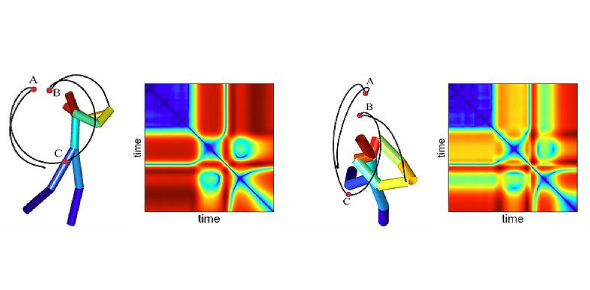# Matrices Self Study Quiz

9 Questions | Attempts: 1806SettingsQuiz on the Self Study topic of Matrices done by Ong Tiet Ho, Hwa Chong Institution, 3S422 as Part III of the June Self Study Modules. Topics tested include the basics of matrices, addition, subtraction and multiplication of matrices.

• 1.
Which of the following is impossible?
• A.

Scalar Multiplication

• B.

Matrix Multiplication

• C.

Scalar Division

• D.

Matrix Division

• 2.
What is an identity matrix and why is it called an identity matrix?
• A.

An identity matrix is a matrix with all diagonal entries as 1 and all non-diagonal entries as 0. It is called an identity matrix as it would give a null matrix as a result when multiplied by other matrix.

• B.

An identity matrix is a matrix with all diagonal entries as 1 and all non-diagonal entries as 0. It is called an identity matrix as it would not change the original matrix when multiplied by it.

• C.

An identity matrix is a matrix with all its entries as 0. It is called an identity matrix as it would give a null matrix as a result when multiplied by any other matrix.

• D.

An identity matrix is a matrix with all its entries as 0. It is called an identity matrix as it would not change the original matrix when multiplied by it.

• 3.
(9  0  1  3  4)           (0  7  3  0)A = (5  7  8  2  4)           (1  3  6  2)      (3  4  2  7  0)     B = (7  8  9  2)                                    (7  4  3  8)                                    (8  3  2  5)Which of the following is possible?
• A.

A + B

• B.

A - B

• C.

A x B

• D.

A / B

• 4.
Which of the following matrix operations is commutative?
• A.

• B.

Subtraction of Matrices

• C.

Multiplication of Matrices

• D.

Division of Matrices

• 5.
Which of the following is not a square matrix?
• A.

(1)

• B.

(5 8) (9 2)

• C.

(4 9) (16 25) (36 49)

• D.

(2 8 7 6 9) (8 7 6 0 3) (7 6 8 3 4) (1 4 7 2 6) (7 8 2 3 0)

• 6.
(8  3  1  2)            (7  8  2   5)A = (9  3  4  8)     B = (4  8  2  11)     X = A + B      (5  6  7  0)            (3  7  1   9)What do x2,3 and x1,4 represent? Give your answer in the form x2,3, x1,4.
• 7.
For addition to be possible, two matrices must have the same __________. For multiplication to be possible, the number of __________ in the first matrix must be the same as the number of __________ in the second matrix. The resultant matrix would have the number of __________ the first matrix has, and the number of ___________ the second matrix has.Fill in the above blanks, with a comma and a space in between succeding answers.
• 8.
A = 3 (1  2  8)     B = (5) (7  4  9)Evaluate A and B and present your answers as A, B with two spacings between each entry in each matrix.
• 9.
A = (4  9  8  2  1)           (2)                                    (9)                              B = (2)                                    (3)                                    (4)Evaluate AB and BA and present your answers as AB, BA.

## Related TopicsBack to top
×

Wait!
Here's an interesting quiz for you.